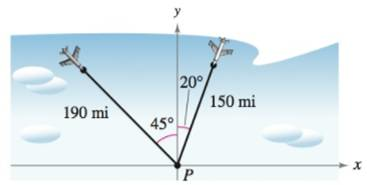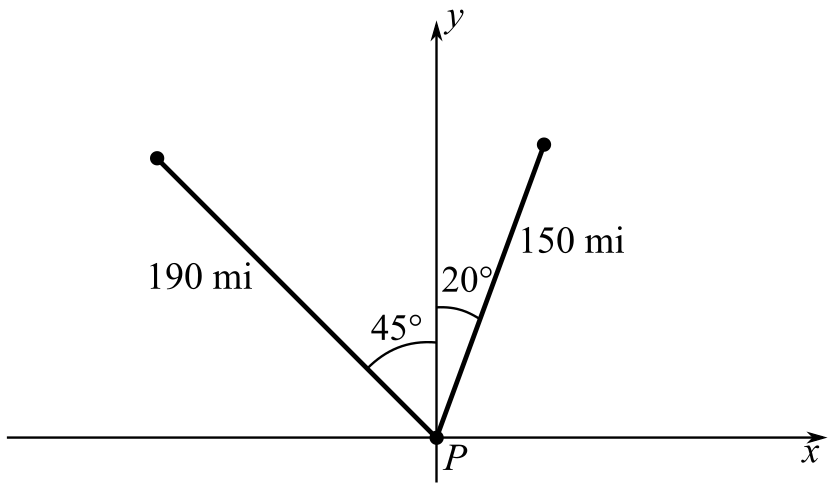Chapter 10, Problem 4PS

Chapter
Section
Textbook Problem

# Flight Paths An air traffic controller spots two planes at the same altitude flying toward each other (see figure). Their flight paths are 20° and 315°. One plane is 150 miles from point P with a speed of 375 miles per hour. The other is 190 miles from point P with a speed of 450 miles per hour.(a) Find parametric equations for the path of each plane where t is the time in hours, with t = 0 corresponding to the time at which the air traffic controller spots the planes.(b) Use the result of part (a) to write the distance between the planes as a function of t.(c) Use a graphing utility to graph the function in part (b). When will the distance between the planes be minimum? If the planes must keep a separation of at least 3 miles, is the requirement met?

(a)

To determine

To calculate: The parametric equations for the path of each plane if

The flight paths are 20° and 315°.

One plane is 150 miles from P with a speed of 375 miles per hour.

The other plane is 190 miles from point P with a speed of 450 miles per hour.

The figure is given below.Explanation

Given:

The flight paths are 20° and 315°.

One plane is 150 miles from P with a speed of 375 miles per hour.

The other plane is 190 miles from point P with a speed of 450 miles per hour.

The given figure is:

Formula used:

Speed formula:

speed=distancetime

Calculation:

Observe from the given figure, that the angle made by one plane with positive x-axis is

90°20°=70°

Distance of the flight from the point P is 150 miles.

Since t=0 it corresponds to the time when the air traffic controller spots the plane. Hence, the distance covered by the plane with a speed of 375 miles per hour is 375t

(b)

To determine

To calculate: The distance between the planes as a function of t if

The parametric equation of one of the planes:

x1=(150375t)cos70°y1=(150375t)sin70°

The parametric equation of another plane:

x2=(190450t)cos135°y2=(190450t)sin45°

(c)

To determine

To graph:

The function y=[(190450t)cos135°(150375t)cos70°]2+[(190450t)sin45°(150375t)sin70°]2 and calculate the minimum distance between the planes and find if the planes keep a separation of at least 3miles.

### Still sussing out bartleby?

Check out a sample textbook solution.

See a sample solution

#### The Solution to Your Study Problems

Bartleby provides explanations to thousands of textbook problems written by our experts, many with advanced degrees!

Get Started

#### In problems 15-22, simplify by combining like terms. 15.

Mathematical Applications for the Management, Life, and Social Sciences

#### Divide: +4800240

Elementary Technical Mathematics

#### Let and g(x) = x + 3. Then (g ∘ h)(x) = ______.

Study Guide for Stewart's Single Variable Calculus: Early Transcendentals, 8th